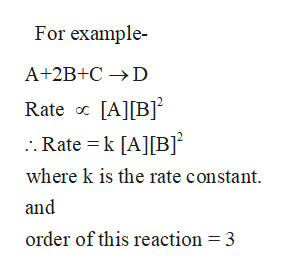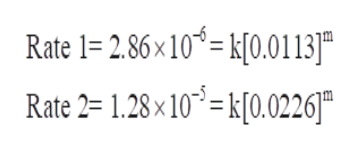Given the following reactionCH3NNCH3(g) ==> C2H6(g) + N2(g)and the measured kinetic data below: [ CH3NNCH3(g) ] MRate M/s0.01132.86 x 10-60.02261.28 x 10-5 what is the order with respect to (each of the) reactants CH3NNCH3(g),?Type your calculated answer for CH3NNCH3(g) here:

Question

Given the following reaction

CH3NNCH3(g) ==> C2H6(g) + N2(g)

and the measured kinetic data below:

[ CH3NNCH3(g) ] M Rate M/s
0.0113 2.86 x 10-6
0.0226 1.28 x 10-5

what is the order with respect to (each of the) reactants CH3NNCH3(g),?

Type your calculated answer for CH3NNCH3(g) here:

Step 1
• The rate law of a reaction expresses the relationship between the concentration of the reactant species and the rate of that chemical reaction.
• And Order is equal to the sum of the exponents of the concentration of reactant species in the rate law.help_outlineImage TranscriptioncloseFor example A+2B+C D Rate [A[B . Rate k [A] [B] where k is the rate constant and order of this reaction = 3 fullscreen
Step 2

For the given reaction , let the order of reaction with respect to CH3NNCH3 be x. Therefore Rate law can be written as-

Rate = k[CH3NNCH3]m where k is the rate constant.

Step 3

The given data is -

 [CH3NNCH3], M Rate M/s 0.0113 2.86 x 10-6 0.0226 1.28 x 10-5

Thus we can write two different equations for rate law and then evaluate them to determine the value of order of th...help_outlineImage TranscriptioncloseRate 1= 2.86 x 10= k[0.0113]| Rate 2= 1.28x10-k[0.0226] fullscreen

Want to see the full answer?

See Solution

Want to see this answer and more?

Our solutions are written by experts, many with advanced degrees, and available 24/7

See Solution
Tagged in

Chemistry